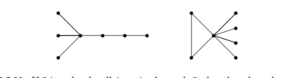# If l, m, n are nonnegative integers

If l, m, n are nonnegative integers with l + m =n ≥ 1, then there exists a connected simple n-vertex graph with l vertices of even degree and m

Since every graph has an even number of vertices of odd degree, and the only simple connected graph with two vertices has both degrees odd, the condition is necessary.To prove sufficiency, we construct such a graph G. If m = 0, let G = Cl (except
G = K1 if l = 1).For m > 0, we can begin with K1,m−1, which has m vertices of odd degree, and then add a path of length l beyond one of the leaves. (Illustration shows l = 3, m = 4.)

Alternatively, start with a cycle of length l, and add m vertices of degree one with a common neighbor on the cycle. That vertex of the cycle has even degree because m is even. Many other constructions also work. It is also possible to prove sufficiency by induction on
n for n ≥ 3, but this approach is longer and harder to get right than an explicit general construction.### Submit Assignment

Fill in the form below or give us a call and we'll contact you. We endeavour to answer all enquiries within 24-48 hours on business days.
[contact-form-7 id="7458"]